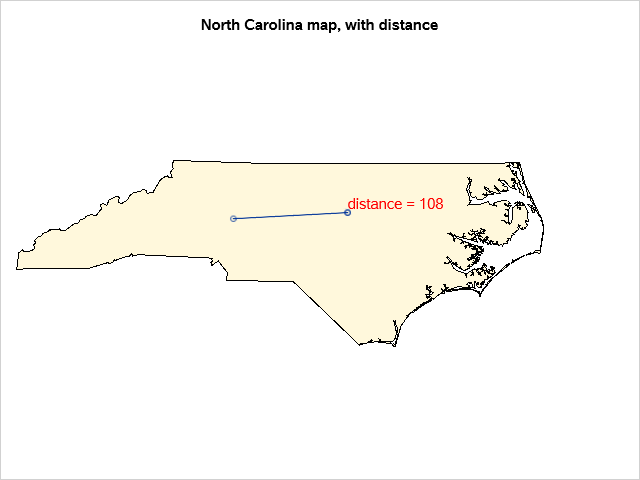## SAS Studio SGMap: Can distance lines between 2 points be plotted on a map in Univ. Ed. SAS Studio

Hello,

I am very new to base SAS, but stumbled upon sgmap and thought that it would be very useful for a research project my department is working on. I would like to plot locations using longitude and latitude data, then calculate and plot distance lines between pairs of locations. Is this possible in University Edition of SAS studio? I have only seen bubble plots without lines using sgmap. I don't currently have access to SAS/graph which I know can do what I want.

Thank you,

KellyP

1 ACCEPTED SOLUTION

Accepted Solutions

## Re: SAS Studio SGMap: Can distance lines between 2 points be plotted on a map in Univ. Ed. SAS Studi

Here is how I programmed the lines and distance labels. I will probably adjust the formatting some more, but I am close to what I want.

``````data maptest;
set maptes.sheet1;
format distance comma10.2;
distance=geodist(lat1, lon1, lat2, lon2, 'M');
Route+1;
DistLabel=catx(" ", round(distance, 0.01),"miles");
run;

data map_data_rotate (keep=year Location lat lon distance route DistLabel latmid lonmid);
set maptest;
format lat lon 8.4;
latmid=(lat1+lat2)/2;
lonmid=(lon1+lon2)/2;
array lata(2) 8 Lat1 Lat2;
array lona(2) 8 Lon1 Lon2;
do i= 1 to 2;
If lata(i) then lat=lata(i);
if lona(i) then lon=lona(i);
if i=1 then location=start_location;
if i=2 then location=Destination;
output;
end;
run;

title Years 2018 and 2019;

proc sgmap plotdata=map_data_rotate;
openstreetmap;
series x=lon y=lat /group=Route ;
scatter x=lonmid y=latmid /group=Route datalabel=distlabel DATALABELPOS=right DATALABELATTRS=(color=green size=8pt);

run;

title Year 2018;
proc sgmap plotdata=map_data_rotate;
openstreetmap;
where year=2018;
series x=lon y=lat /group=Route ;
scatter x=lonmid y=latmid /group=Route datalabel=distlabel DATALABELPOS=right DATALABELATTRS=(color=green size=8pt);
run;

title Year 2019;
proc sgmap plotdata=map_data_rotate;
openstreetmap;
where year=2019;
series x=lon y=lat /group=Route ;
scatter x=lonmid y=latmid /group=Route datalabel=distlabel DATALABELPOS=right DATALABELATTRS=(color=green size=8pt);
run;
title;``````

5 REPLIES 5

## Re: SAS Studio SGMap: Can distance lines between 2 points be plotted on a map in Univ. Ed. SAS Studi

SAS Academics on Demand supports SAS/Graph if you need it. Same restrictions as SAS UE.

## Re: SAS Studio SGMap: Can distance lines between 2 points be plotted on a map in Univ. Ed. SAS Studi

Thank you! This is very good to know!

## Re: SAS Studio SGMap: Can distance lines between 2 points be plotted on a map in Univ. Ed. SAS Studi

Here is one way to do it, using Proc SGmap in the latest version of SAS (9.4m6) ...

data my_map; set mapsgfk.us_states (where=(statecode='NC'));
run;
data my_map_data; set mapsgfk.us_states_attr (where=(statecode='NC'));
run;

data points;
lat=35.73; long=-80.68; output;
lat=35.82; long=-78.76; output;
distance='distance = '||trim(left(put(geodist(35.73, -80.68, 35.82, -78.76, 'DM'),comma5.0))); output;
run;

ods path(prepend) work.templat(update);
proc template;
define style styles.nc_style;
parent=styles.htmlblue;
class graphcolors / 'gdata1'=cornsilk;
end;
run;

ods html style=nc_style;

title1 "North Carolina map, with distance";
proc sgmap maprespdata=my_map_data mapdata=my_map plotdata=points noautolegend;
choromap statecode / mapid=statecode;
scatter x=long y=lat;
series x=long y=lat;
text x=long y=lat text=distance / position=topright textattrs=(color=red size=11pt);
run;## Re: SAS Studio SGMap: Can distance lines between 2 points be plotted on a map in Univ. Ed. SAS Studi

Thank you! This definitely helped me figure out my program!

## Re: SAS Studio SGMap: Can distance lines between 2 points be plotted on a map in Univ. Ed. SAS Studi

Here is how I programmed the lines and distance labels. I will probably adjust the formatting some more, but I am close to what I want.

``````data maptest;
set maptes.sheet1;
format distance comma10.2;
distance=geodist(lat1, lon1, lat2, lon2, 'M');
Route+1;
DistLabel=catx(" ", round(distance, 0.01),"miles");
run;

data map_data_rotate (keep=year Location lat lon distance route DistLabel latmid lonmid);
set maptest;
format lat lon 8.4;
latmid=(lat1+lat2)/2;
lonmid=(lon1+lon2)/2;
array lata(2) 8 Lat1 Lat2;
array lona(2) 8 Lon1 Lon2;
do i= 1 to 2;
If lata(i) then lat=lata(i);
if lona(i) then lon=lona(i);
if i=1 then location=start_location;
if i=2 then location=Destination;
output;
end;
run;

title Years 2018 and 2019;

proc sgmap plotdata=map_data_rotate;
openstreetmap;
series x=lon y=lat /group=Route ;
scatter x=lonmid y=latmid /group=Route datalabel=distlabel DATALABELPOS=right DATALABELATTRS=(color=green size=8pt);

run;

title Year 2018;
proc sgmap plotdata=map_data_rotate;
openstreetmap;
where year=2018;
series x=lon y=lat /group=Route ;
scatter x=lonmid y=latmid /group=Route datalabel=distlabel DATALABELPOS=right DATALABELATTRS=(color=green size=8pt);
run;

title Year 2019;
proc sgmap plotdata=map_data_rotate;
openstreetmap;
where year=2019;
series x=lon y=lat /group=Route ;
scatter x=lonmid y=latmid /group=Route datalabel=distlabel DATALABELPOS=right DATALABELATTRS=(color=green size=8pt);
run;
title;``````

Discussion stats
• 5 replies
• 773 views
• 7 likes
• 3 in conversation